##Dividend Regressions: January 2020

Variables used in the regression

1. Dividend Yield = Dividends per share in most recent year/ Current Stock Price
2. Dividend Payout Ratio = Dividends / Net Income
3. Beta: Regression or Bottom up beta
4. Expected Growth in EPS over next 5 years = Consensus analyst estimate (or your own) of expected growth in EPS . If you don't have an analyst estimate, use your own estimate of expected growth.
5. Market Debt to Capital = Debt/ (Debt + Market Value of Equity): If you have market value for debt, use it. If not, use book value of debt and market value of equity.

US Regression: Dividend Yield### US Regression Output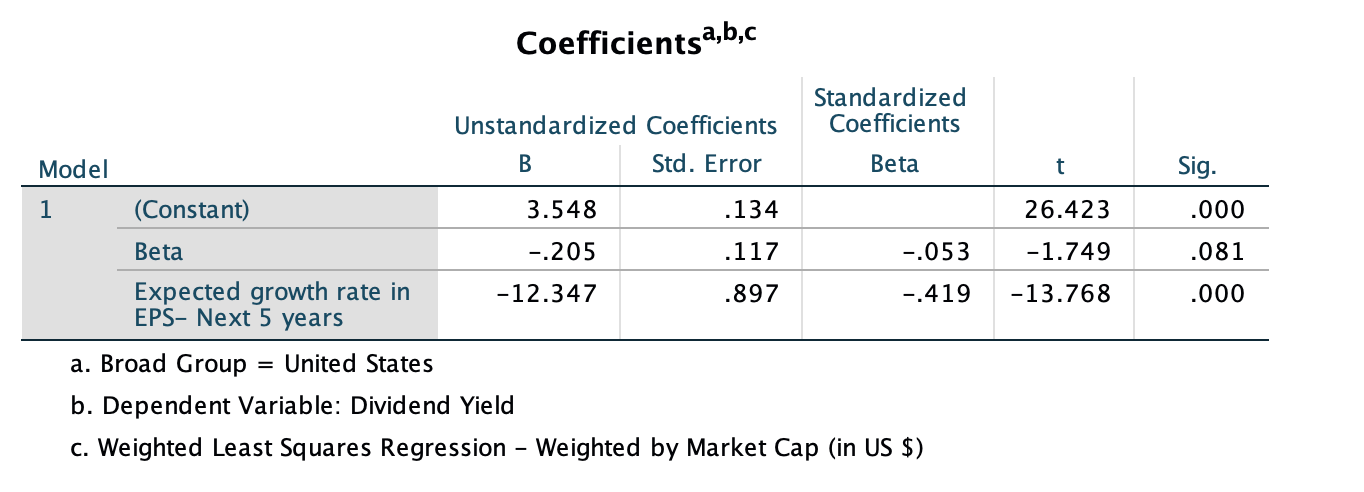US Regression: Dividend Payout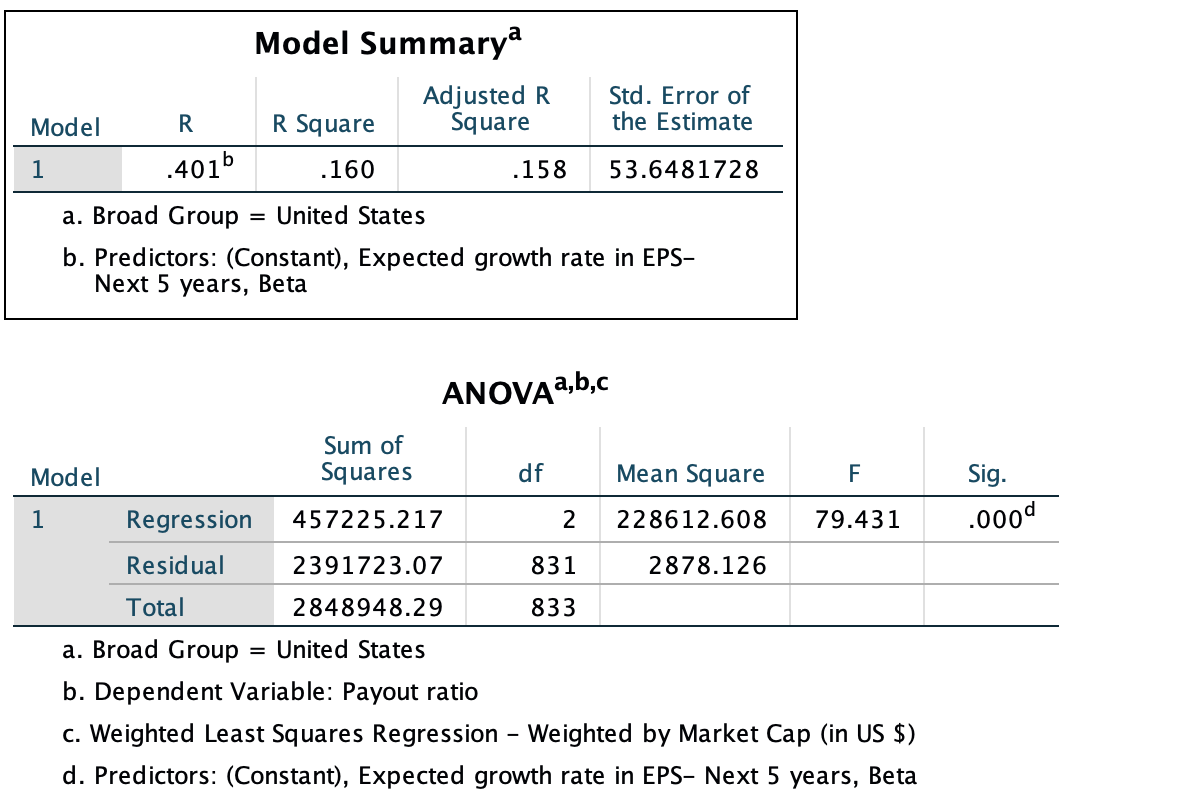### Regression Output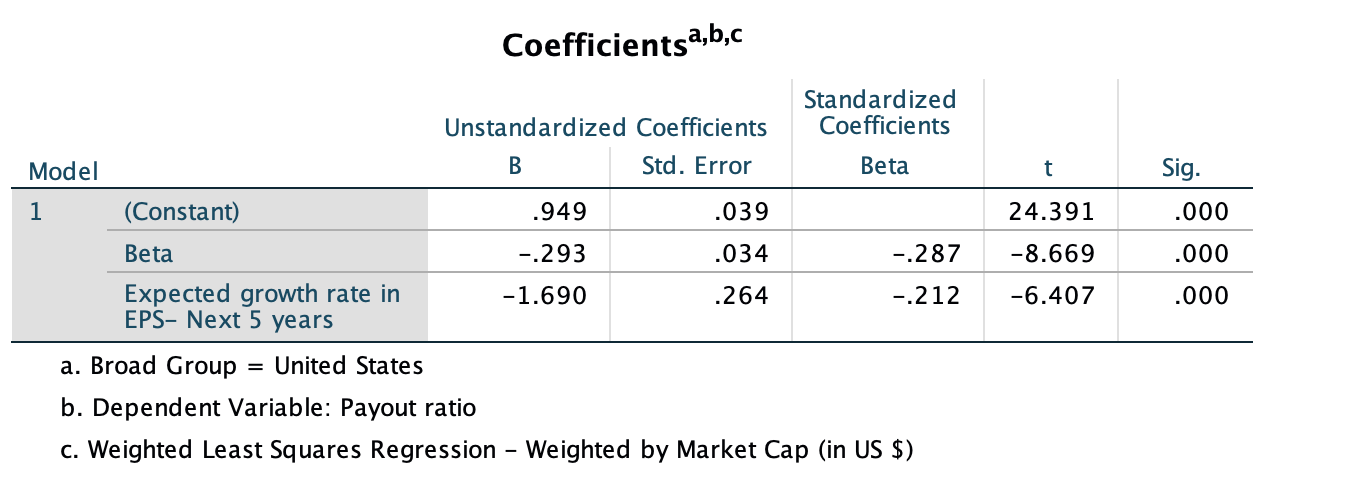Global Regression: Dividend Yield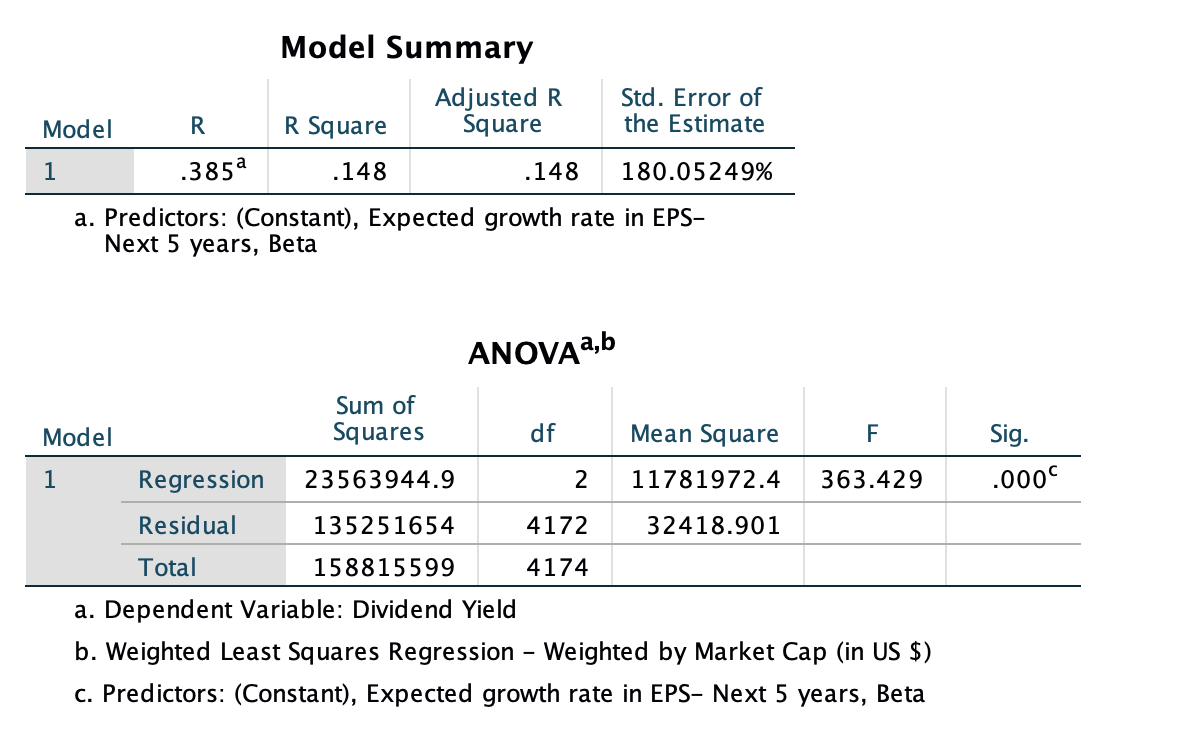### Regression Output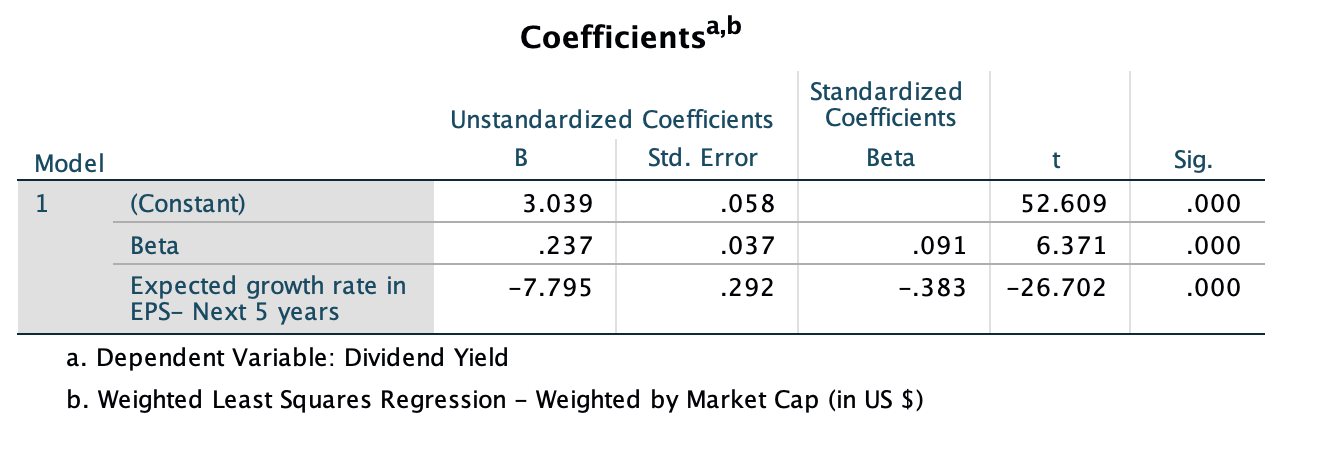Global Regression: Dividend Payout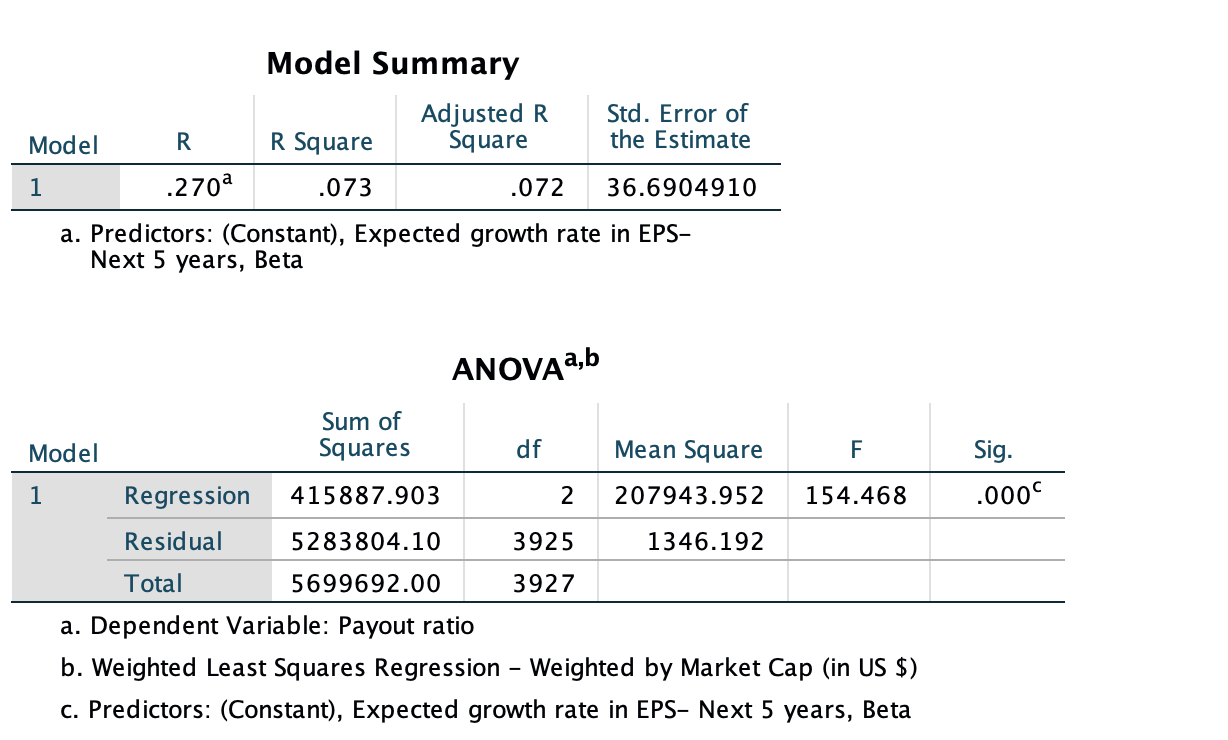### Regression Output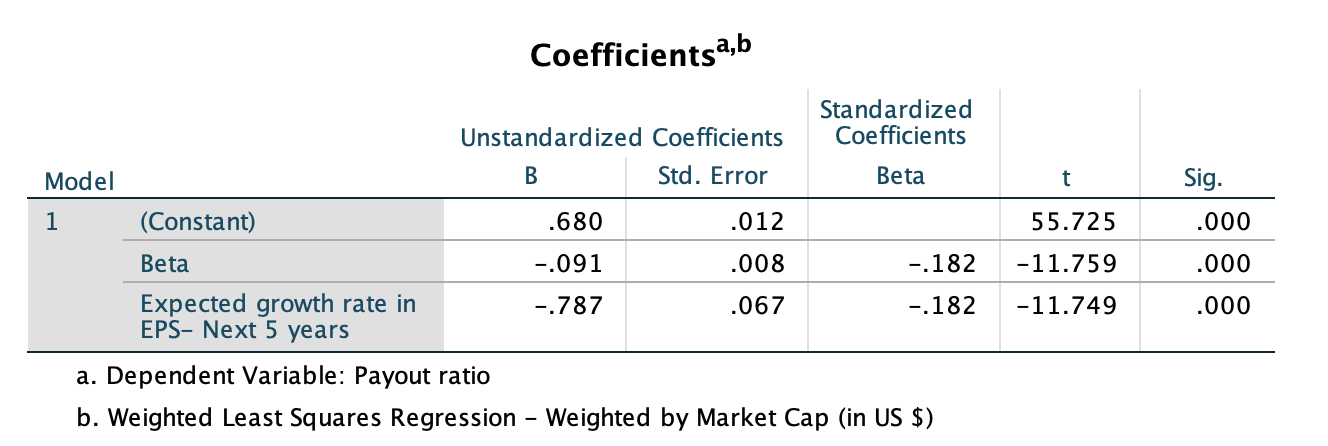• How do I use this regression?

Assume that you want to estimate the dividend payout ratio for a firm with the following characteristics, using the US regression:

Regression beta = 1.20

Expected Growth in EPS over next 5 years = 10%

• Predicted Values
• Expected Dividend Yield =3.548 - 0.205 (1.20) -12.347 (.10) = 2.06 or 2.06%
• Expected Dividend payout ratio = 0.949  - .293 (1.20)  - 1.69 (.10)  = .42 or 42%

If your predicted value is less than zero, your predicted dividend payout ratio is zero.